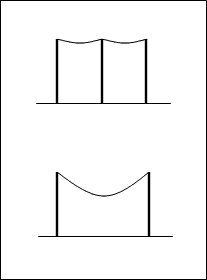# Catenary Correction formula (1 Viewer)

### Users Who Are Viewing This Thread (Users: 0, Guests: 1)

#### Tsmith

Sag formula

When measuring a distance with a 100 foot steel tape supported at both ends the sag formula needs to be applied to correct the distance. Does anybody know why an overall distance does not work in this sag formula? For example If you measure a distance of 735 feet you would have to apply the formula eight times once for each set of measurements. Seven for 100 feet and once for 35 feet and add the eight results. Why does the formula not work if you enter 735 feet?

Cs = (-w squared) (L cubed) / 24 (P squared)

Cs = correction for sag
w = weight per unit length of the tape (pounds per foot)
l = length of the suspended section of tape in feet
P= tension applied to the tape

#### PhanthomJay

Homework Helper
Gold Member
It would work IF your tape was 735 feet long! (and the sags were relatively small compared to the span; the formula you site is the for the parabolic approximation of the more exact catenary curve, and works quite well for small sag/span ratios).
But you only have a 100 foot tape, and the corrections for sag must be made each time for 8 times. This is because the sag in the tape varies as the square of the span, such that the correction must be made for each measurement. The correction for sag, for a 100 foot length, added up over 7 times for a 700 foot measurement,will be much less than the correction factor for a full length 700 foot tape over that distance.
Welcome to PF!

#### Redbelly98

Staff Emeritus
Homework Helper
A quick answer is that the amount of sag is not simply proportional to the length of tape. So for example, a 200 foot tape sags more than twice as much as a 100 foot tape.

Also, a picture is worth a thousand words:#### Bob S

Solving the exact situation of a cable supporting its own weight (uniform catenary problem) over a horizontal distance x:

In this case, the slope of the cable dy/dx as a function of the horizontal distance x from the center is

dy/dx = sinh(x/c) where c = T/w,

T = horizontal tension (pounds) and w = cable weight/foot (pounds per ft.)

Using horizontal distance x and cable length L, the cable length is given by integrating

L = int{ [1 + sinh2(x/c)]1/2} dx from -x/2 to +x/2

L = int{cosh(x/c)} dx from -x/2 to x/2 = 2c sinh(x/c)0x/2 = 2c sinh(x/2c)

Inverting L and x, we get x as a function of L (the measuring tape length)

x = 2c sinh-1(L/2c)

So using measuring tape length L = 100 ft, T = 100 pounds (horizontal tension)
and w = 0.1 pounds per foot, and c = T/w = 1000 feet.

We get for the horizontal (line of sight) distance x = 99.9584 feet.

So in this case for a measured distance using stretched 100 foot tape, the actual horizontal distance is 99.9584 feet. Thus the actual distance is 0.0416 feet shorter than the 100 foot measuring tape. For 700 feet (7 x 100 ft measurements), the actual distance x is 699.7088 feet. adding 34.9982 feet for the final 35 foot measurement, we get a straight line distance of 734.987 feet.

Last edited:

#### PhanthomJay

Homework Helper
Gold Member
You get just about exactly the same value using Tsmith's equation which is based on the parabolic approximation of the catenary curve (y=wl^2/8(T), and delta L = 8(y)^2/3L, thus C_f = w^2(L^3)/24T^2), which is a heck of a lot easier. When sags are small compared to spans (less than a few percent), the approximation works extremely well.

#### Bob S

Although it is always nice to find an adequate approximation like the parabola for suspension bridges, the accuracy of the approximation is not known until the exact equation is solved. Besides, isn't finding the exact solution a challenge?

#### PhanthomJay

Homework Helper
Gold Member
I suppose if you have a calculator with the hyperbolic functions, it's not such a big deal; but when measuring with a tape measure, the accuracy is only as good as your assumed value for tension (and temperature) which could be way off. I learned the correction factor back in the days when all we had were slide rules. I now like the laser measurements instead. No challenge!

#### Tsmith

Thanks for you responses. I work in the survey field. I understood why it would not work but did not have the background in physics to explain it.

### The Physics Forums Way

We Value Quality
• Topics based on mainstream science
• Proper English grammar and spelling
We Value Civility
• Positive and compassionate attitudes
• Patience while debating
We Value Productivity
• Disciplined to remain on-topic
• Recognition of own weaknesses
• Solo and co-op problem solving